Courses
Courses for Kids
Free study material
Free LIVE classes
More

# Protractor Scale: How to Read and Use It## Overview of Protractor Scale

Protractors are useful, clear plastic tools for everyday life; we all have seen the protractor in the geometry box. They can be used for measuring different types of angles. It is a device used to measure and plot angles from 0 to 180 degrees, clockwise and anticlockwise. It has a straight edge with one or more semicircles on the edges.

The protractor has a scale usually marked with degrees or radians (any angle in minutes). It can be used for measuring angles in a plane and three-dimensional space. The protractor scale can measure angles to find the area of a triangle, the volume of a cylinder, and so on. We will be looking at a protractor scale, a scale in maths, how to read a protractor and how to use a protractor with examples in this article.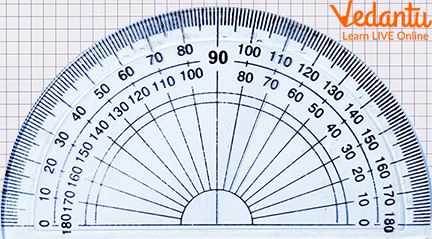A Common Protractor

## What Is a Protractor?

A protractor is a type of measuring tool that helps us find angles. It has two parts, a straightedge and a curved ruler. A line on the protractor represents the angle we are trying to find. Protractors are used in many different maths problems.

A general protractor consists of an inner and outer scale, graduated from 0 degrees to 180 degrees. It is a tool that helps us draw a line or shape using two different angles. It’s beneficial in teaching kids how to use angles and draw shapes. The tool is also helpful for artists, engineers, architects, and many more who need to measure angles. They use different kinds of protractors for accurate measurement of angles.

## 360-degree Protractor

A 360-degree protractor is a tool used to measure angles in a circle. It has a flat and circular surface and can be used for drawing circles. It is also used in geometry.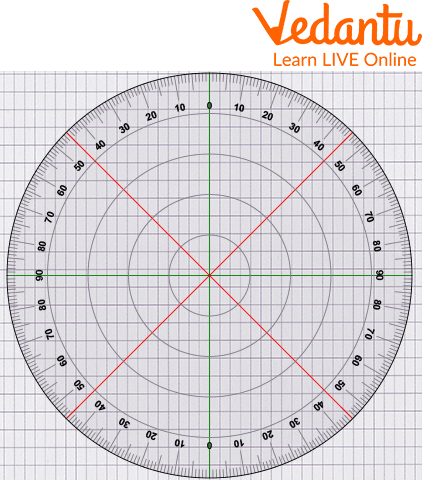A 360-degree Protractor

## What is Scale in Maths?

Scale in Mathematics is a range of levels or numbers used to measure numbers. Here, it is simply the angle marking from 0 degrees to 180 degrees in a general protractor.

## How to Read and Use a Protractor

While measuring through a protractor, the measure is usually in degrees. If the angle is on the left side of the protractor, we use the outer edge measurement, and if the angle is on the right side of the protractor, we use the inner edge measurements.

The steps to measure and read the angle are as follows:

Step 1: Place the protractor's centre on the angle's vertex.

Step 2: Superimpose one side of the angle with the zero line of the protractor.

Step 3: The angle equals the number of degrees crossed on the protractor.

Let us look at an example to read and measure the angle of 60 degrees.

Step 1: Align the protractor with the ray OB as shown below. Start reading from the \$0^{\circ}\$ mark on the bottom-right of the protractor.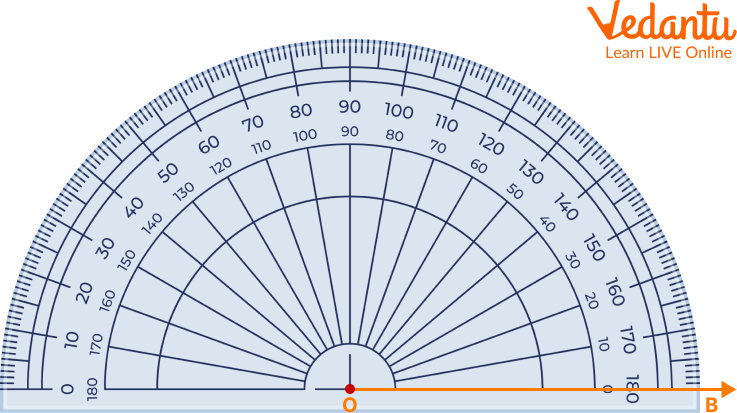Step 2: The angle measure is the number on the protractor that coincides with the second ray. Measure the angle using the number on the lower arc of the protractor.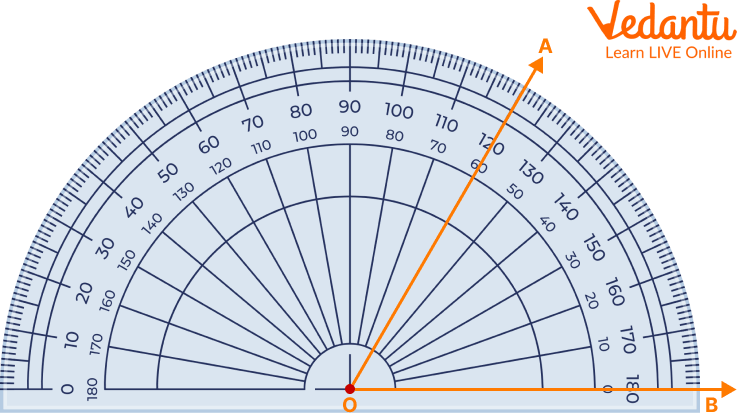Protractor Showing an Angle 60 Degree

Therefore, \$\angle A O B=60^{\circ}\$. Since the measure is greater than \$0^{\circ}\$ and lesser than \$90^{\circ}\$, we can say that \$\angle A O B\$ is an acute angle.

This way, we can read and use the protractor to measure the angle.

## Solved Example

Q1. Find the measure of the angle given in the picture below.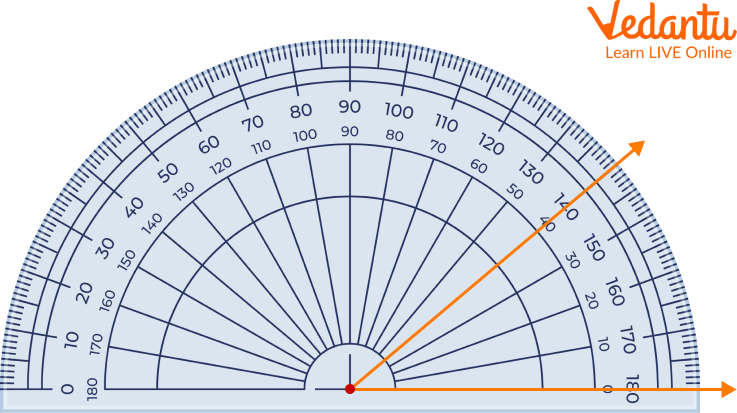Solved Example

Ans: First, place the protractor's centre perfectly on the line.

Now, look at the inner scale of the right side.

Then, check the angle passing through the line.

We get 40 degrees as the answer.

## Practice Questions

Identify the angle measures.

1. The measure of the angle is _____ degrees.

Ans: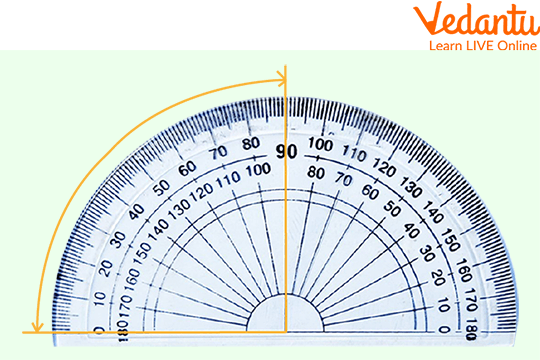Practice Questions

Ans: 90 degrees

2. The measure of the angle is _____ degrees.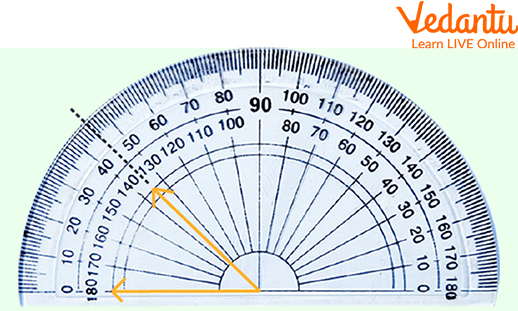Practice Question

Ans: 135 degrees

## Summary

A protractor is a measuring instrument for measuring angles, typically made of transparent plastic or glass. Some protractors are simple half-discs or full circles. More advanced protractors, such as the bevel protractor, have one or two swinging arms, which can be used to help measure the angle. A protractor is a great tool for measuring angles in various mathematical problems.

They are also excellent for use in geometry, measuring the slope of a line, and calculating different volumes and areas. There are two types of protractors: semi-circular (0 degrees to 180 degrees) and circular (0 degrees to 360 degrees). Engineers use these to create technical designs for different construction sites.

Last updated date: 28th Sep 2023
Total views: 91.2k
Views today: 0.91k

## FAQs on Protractor Scale: How to Read and Use It

Q1. Who invented the first protractor?

Ans: The first protractor was invented by 19th-century physicist Philip von Jolly.

Q2Why are there two scales on a protractor?

Ans: There are two scales on a protractor because the two scales make it easy for us to measure angles facing different ways.

Q3. What is the least count on a protractor?

Ans: 1 degree is the least count on a protractor.